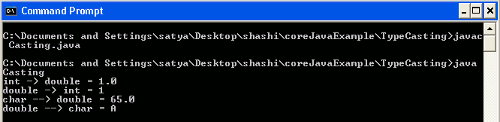Tutorials

# Type casting in java

Type casting is used in Java for converting one type into another type. For example you can typecast string representation of number into int data type. This tutorial explains type casting with example program.

Type casting is used in Java for converting one type into another type. For example you can typecast string representation of number into int data type. This tutorial explains type casting with example program.

## Type casting in java

In this section you will learn about type casting in java. Type casting is to convert one type to another type. Java support two types of casting; primitive type and reference type casting. Reference type casting is nothing but  casting one object type to another object type. In simple word type casting is a conversion of one data type to another data type  it could be class or interface. Primitive Data type conversion are of  two types they are as follows:

• Implicit casting : Implicit casting is also called widening because when you try to assign a lower data type to higher data type it is called widening. In more simple word a data type of lower size is assigned to data type of higher size and it is an automatic conversion done by JVM.
• ```int a=10;  // occupies 4 byte
double b=a; // occupies 8 byte
System.out.prinln(b); // prints 10.0
```
• Explicit Casting : A data type of higher size is assigned to data type of  lower size is called narrowing or explicit casting. This is not done by JVM implicitly  casting is done by programmer explicitly, higher size is narrowed to lower size.
• ```    double a=12.3  // occupies 8 byte
int b=a;    // compilation error
```

In the above code double type value is assigned do lower type int , JVM will not do it implicitly so it raises compilation error. This could be written as follows :

``` double a = 12.5;
int b = (int)a;  // explicit casting a double type cast to int type   ```

Reference type casting : In java type casting is to cast one type, a class or interface to another type i.e. another class or interface. Since java is a object oriented programming language which support inheritance, it is possible that a super class object is referring to a sub class object but there is no any way that compiler will know that super class variable is pointing to a sub class object, it means you cannot directly call a method declared in sub class. For that you need to be cast object . Suppose there is class A which is super class and B is a sub class to A and it look like as :

class A
|
class B extends A

Now look at following code :

``` A a=new B(); // reference type of base class pointing to derived type.
B b=a; // compile time error require casting
B b=(B)a; // type casting base to derived
```

In above code casting object of derived class b  into base class A it will throw an classCastException if  a  is not an object of derived class.

Example :  Code  for type casting

```public class Casting {

public static void main(String args[])
{
int a=1;
double b;
char d='A';
b=a;      // converting int to double(Implicit conversion)

System.out.println("int -> double = "+b);
a=(int)b; //Explicit conversion

System.out.println("double -> int = "+a);
b=d;       //Implicit conversion
System.out.println("char --> double = "+b);

d=(char)b;   // Explicit conversion to char
System.out.println("double --> char = "+d);
}
}```

Output :  After executing the above program.